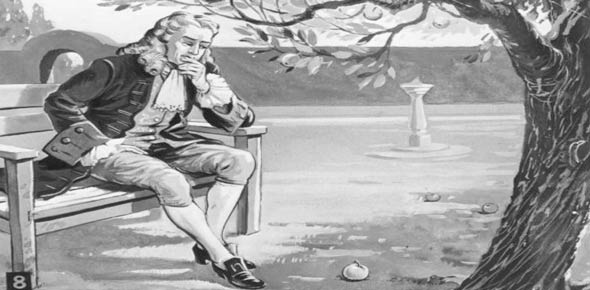# The Quiz Of Three Laws Of Motion

Approved & Edited by ProProfs Editorial Team
The editorial team at ProProfs Quizzes consists of a select group of subject experts, trivia writers, and quiz masters who have authored over 10,000 quizzes taken by more than 100 million users. This team includes our in-house seasoned quiz moderators and subject matter experts. Our editorial experts, spread across the world, are rigorously trained using our comprehensive guidelines to ensure that you receive the highest quality quizzes.
| By Codepacker
C
Codepacker
Community Contributor
Quizzes Created: 1 | Total Attempts: 464
Questions: 6 | Attempts: 464SettingsThis is the Quiz of Newton's three laws of motion.

• 1.

### What are newton's first law of motion?

• A.

An object at rest stays at rest unless an force acts apon it.

• B.

F=MA

• C.

For every action there is always a oppsite reaction.

A. An object at rest stays at rest unless an force acts apon it.
Explanation
Newton's first law of motion states that an object at rest will remain at rest unless acted upon by an external force. This means that if no force is applied to an object, it will continue to stay still. However, if a force is exerted on the object, it will start to move. This law is also known as the law of inertia, which describes how objects tend to resist changes in their state of motion.

Rate this question:

• 2.

### What is newton's third law?

That every action has a oppsite reaction
Explanation
Newton's third law states that for every action, there is an equal and opposite reaction. This means that whenever an object exerts a force on another object, the second object exerts an equal and opposite force back on the first object. This law applies to all types of forces, whether they are contact forces or forces acting at a distance. It is a fundamental principle in physics and helps explain how objects interact with each other.

Rate this question:

• 3.

### What is the formula for newton's second law?

F=MA
Ma=F
Explanation
The correct formula for Newton's second law is F=MA, which means that the force acting on an object is equal to its mass multiplied by its acceleration. The alternative option, Ma=F, is incorrect as it represents the same equation but in a different format.

Rate this question:

• 4.

### What is the scientist that came up with the three laws of motion?

• A.

Issac Newton

• B.

Gorge Washington

A. Issac Newton
Explanation
Isaac Newton is the scientist who came up with the three laws of motion. His laws are fundamental principles in physics that describe the relationship between the motion of an object and the forces acting upon it. Newton's laws of motion are widely used and have been instrumental in advancing our understanding of the physical world.

Rate this question:

• 5.

### True or False? The Greater the force the greater the motion of the object moving?

• A.

True

• B.

False

A. True
Explanation
The statement is true because according to Newton's second law of motion, the acceleration of an object is directly proportional to the net force acting on it. Therefore, if the force acting on an object increases, its acceleration and consequently its motion will also increase.

Rate this question:

• 6.

### True or False?  Newton was the first one to come up with this theory? The 3 laws of motion

• A.

False

• B.

True

B. True
Explanation
Newton was indeed the first one to come up with the theory of the 3 laws of motion. These laws, also known as Newton's laws of motion, were first published by Sir Isaac Newton in his book "Philosophiæ Naturalis Principia Mathematica" in 1687. They are fundamental principles that describe the relationship between a body and the forces acting upon it. The laws explain how objects move, the causes of motion, and the effects of forces. Newton's laws of motion laid the foundation for classical mechanics and revolutionized our understanding of the physical world.

Rate this question:

Related TopicsBack to top